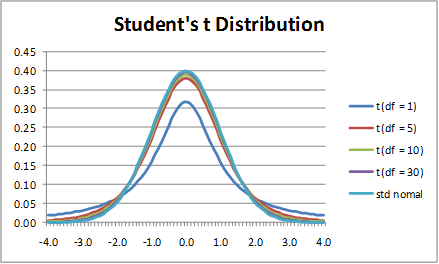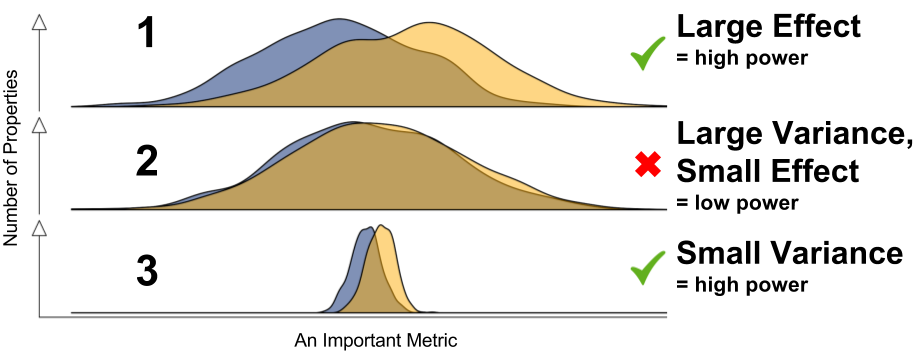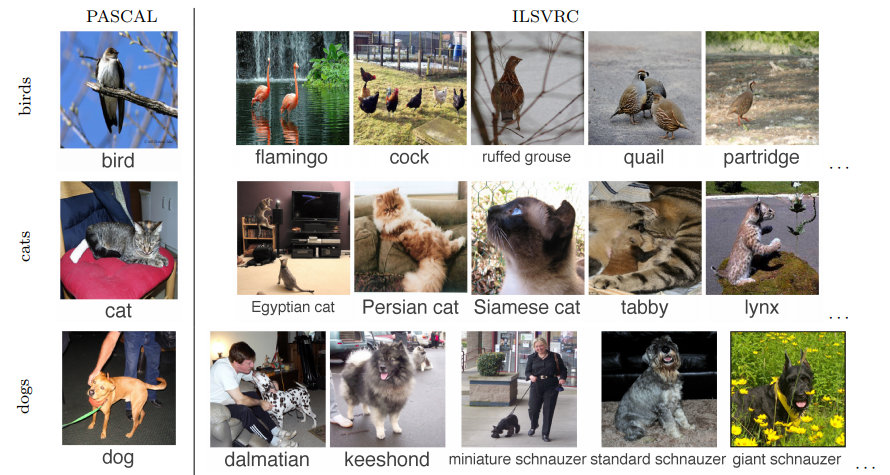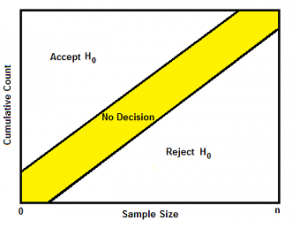Python Code for TTEST and ANOVATTEST What is t-score? The t score is a ratio between the difference between two groups and the difference within the groups. Types of t-tests? There are three main types of t-test: 1. An Independent Samples t-test compares the means for two groups. 2. A Paired sample t-test compares means from the same group at different times (say, one year apart). 3. A One sample t-test tests the mean of […]

Variance Reduction Techniques to Improve Power of the TestImproving the Power of our experiment¶ Now that we understand the system we are dealing with, we can ask the question: how can we increase the detectable effect size of our experiments? We are left with a few options: Increase the effect size Increase the sample size Decrease the variance Increasing the effect size may […]

Central Limit Theorem, Violations & RemedyNormal Distribution About 68% of values drawn from a normal distribution are within one standard deviation σ away from the mean; about 95% of the values lie within two standard deviations; and about 99.7% are within three standard deviations. This fact is known as the 68-95-99.7 (empirical) rule, or the 3-sigma rule. What is Central Limit Theorem (CLT)? In […]

Multi-Armed Bandits and Contextual-BanditMulti-armed bandit uses machine learning algorithms to minimize opportunity costs and minimize regret. They’re more efficient because they move traffic towards winning variations gradually, instead of forcing you to wait for a “final answer” at the end of an experiment. They’re faster because samples that would have gone to obviously inferior variations can be assigned to […]

Multivariate Tests (Orthogonal Design)Because resources are limited, it is very important to get the most information from each experiment you do. Well-designed experiments can produce significantly more information and often require fewer runs than haphazard or unplanned experiments. Also, a well-designed experiment will ensure that you can evaluate the effects that you have identified as important. As a […]

Using Google’s Convolutional Neural Networks (CNN) for Image RecognitionConvolutional neural networks are the state of the art technique for image recognition-that is, identifying objects such as people or cars in pictures.   We call this a “deep neural network” because it has more layers than a traditional neural network. How Convolution Works Instead of feeding entire images into our neural network as one […]

Sequential Probability Ratio TestWhat is a Sequential Probability Ratio Test? A sequential probability ratio test (SPRT) is a hypothesis test for sequential samples. Sequential sampling works in a very non-traditional way; instead of a fixed sample size, you choose one item (or a few) at a time, and then test your hypothesis. You can either: Reject the null hypothesis (H0) in favor of […]

Sampling and Sampling BiasIn statistics, sampling bias is a bias in which a sample is collected in such a way that some members of the intended population are less likely to be included than others. It results in a biased sample, a non-random sample of a population (or non-human factors) in which all individuals, or instances, were not equally likely to have been selected.If this […]

Dealing with Imbalanced DataWhat is Imbalanced Data? Imbalanced data typically refers to a problem with classification problems where the classes are not represented equally. Imbalanced data example: the red points are greatly outnumbered by the blue. In reality, datasets can get far more imbalanced than this. Here are some examples: About 2% of credit card accounts are […]